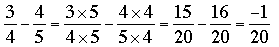SEARCH HOMEMath Central Quandaries & QueriesQuestion from Lavada, a student: 1/3-5/6=1/x How do I solve this problem.Let me try this one

Solve for x if 3/4 - 4/5 = 2/x

First I would find a common denominator and add the two fractions on the left side. A common denominator is 20 so for the left side I getHence the equation becomes

-1/20 = 2/x

To make the fraction on the right equivalent to the fraction on the left I would need x to be -40 since 2/-40 = 1/-20 = -1/20. Hence x = -40.